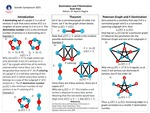## Stander Symposium Projects

#### Title

Domination and F-Domination

#### Presenter(s)

Noah Jacob Kilps

This poster reflects research conducted as part of a course project designed to give students experience in the research process. Course: MTH 466

#### Description

This project is for MTH 466, Graph Theory and Combinatorics. A graph is a mathematical object that consists of two sets, a set of vertices and a set of edges in which an edge between two vertices denotes a relationship between those vertices. A dominating set of a graph G is a set of vertices S such that every vertex of G is a neighbor of some vertex in S. The domination number is the minimum number of vertices in a dominating set S. Let F be a graph whose vertex set is partitioned into two sets: blue vertices and red vertices. Let v be a designated blue vertex of F. An F-coloring of a graph G is a red-blue coloring of the vertices of G in which every blue vertex u belongs to a copy of F rooted at v. The F-domination number is the minimum number of red vertices in an F-coloring of G. We will compare the properties of the domination number and the F-domination number.

4-22-2021

#### Project Designation

Course Project

Aparna W. Higgins

Mathematics

#### Keywords

Stander Symposium Posters, College of Arts and SciencesCOinS